﻿ Python 实现批量分类汇总并保存xlsx文件 - 永恒君的百宝箱

# Python 实现批量分类汇总并保存xlsx文件

931℃上一篇文件用VBA介绍了如何实现一键按列分类汇总并保存单独文件，代码有几十行，而且一旦数据量多了，效果可能不尽如人意。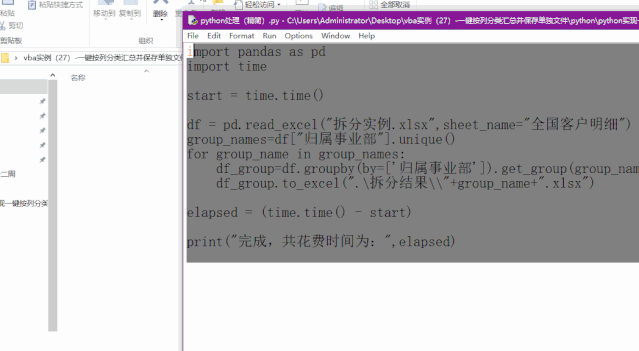### 思路与代码详解

1、这里使用的是python中的pandas数据处理库，这个是在数据处理界非常牛逼的一个工具库，使用之前需要导入库。

``````import pandas as pd
``````

2、读取excel的数据。读取”拆分实例.xlsx”这个excel中,sheet名字为”全国客户明细”的数据，将读取的内容赋值给df。

``````df = pd.read_excel("拆分实例.xlsx",sheet_name="全国客户明细")
``````

3、获取“归属事业部”列的种类数，使用pandas库的unique方法，将所有事业部的名字赋值给变量group_names。

``````group_names=df["归属事业部"].unique()
``````

4、将某个事业部的数据整行提取出来保存成xlsx文件，并按事业部的名字进行命名。

``````df_group=df.groupby(by=['归属事业部']).get_group(group_name).reset_index(drop=True)
df_group.to_excel(".\拆分结果\\"+group_name+".xlsx")
``````

5、遍历每一个事业部，进行同样的操作。

``````for group_name in group_names:
``````

``````import pandas as pd
import time

start = time.time()

group_names=df["归属事业部"].unique()
for group_name in group_names:
df_group=df.groupby(by=['归属事业部']).get_group(group_name).reset_index(drop=True)
df_group.to_excel(".\拆分结果\\"+group_name+".xlsx")

elapsed = (time.time() - start)

print("完成，共花费时间为：",elapsed)
``````

### 优化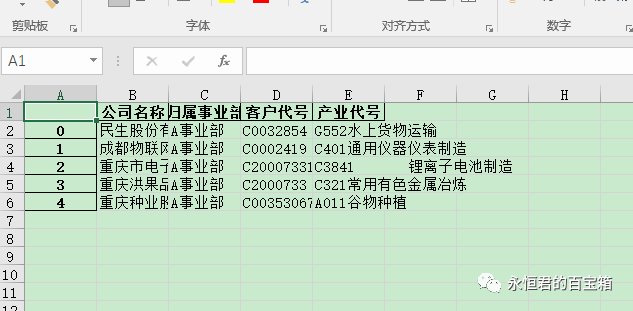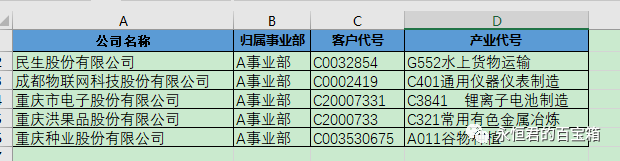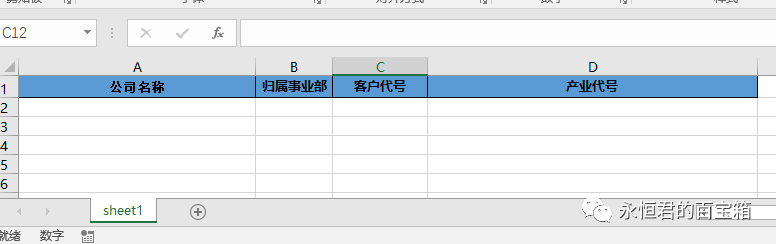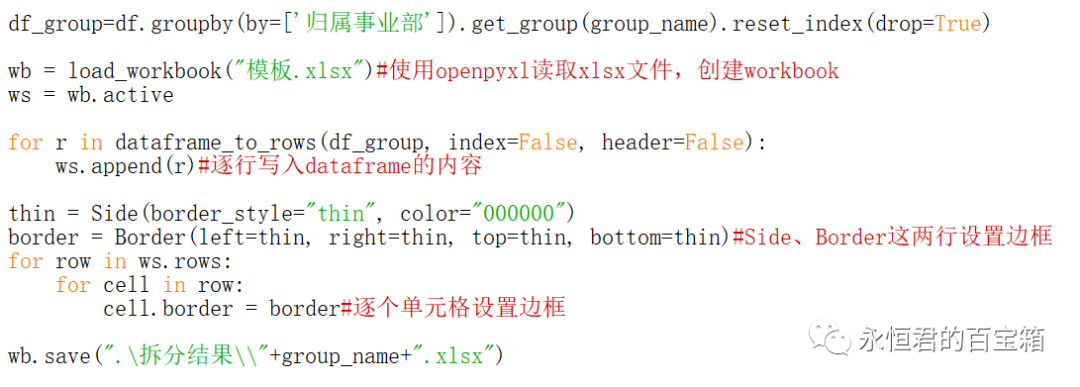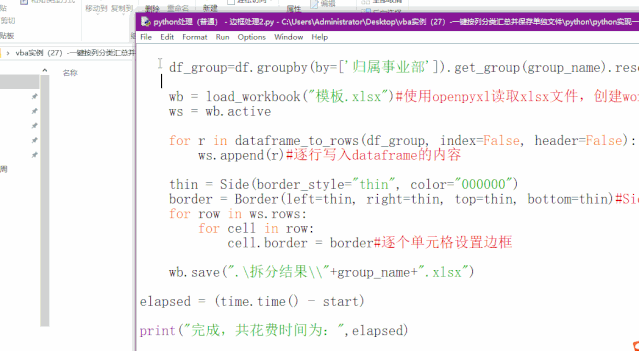anyShare分享到：